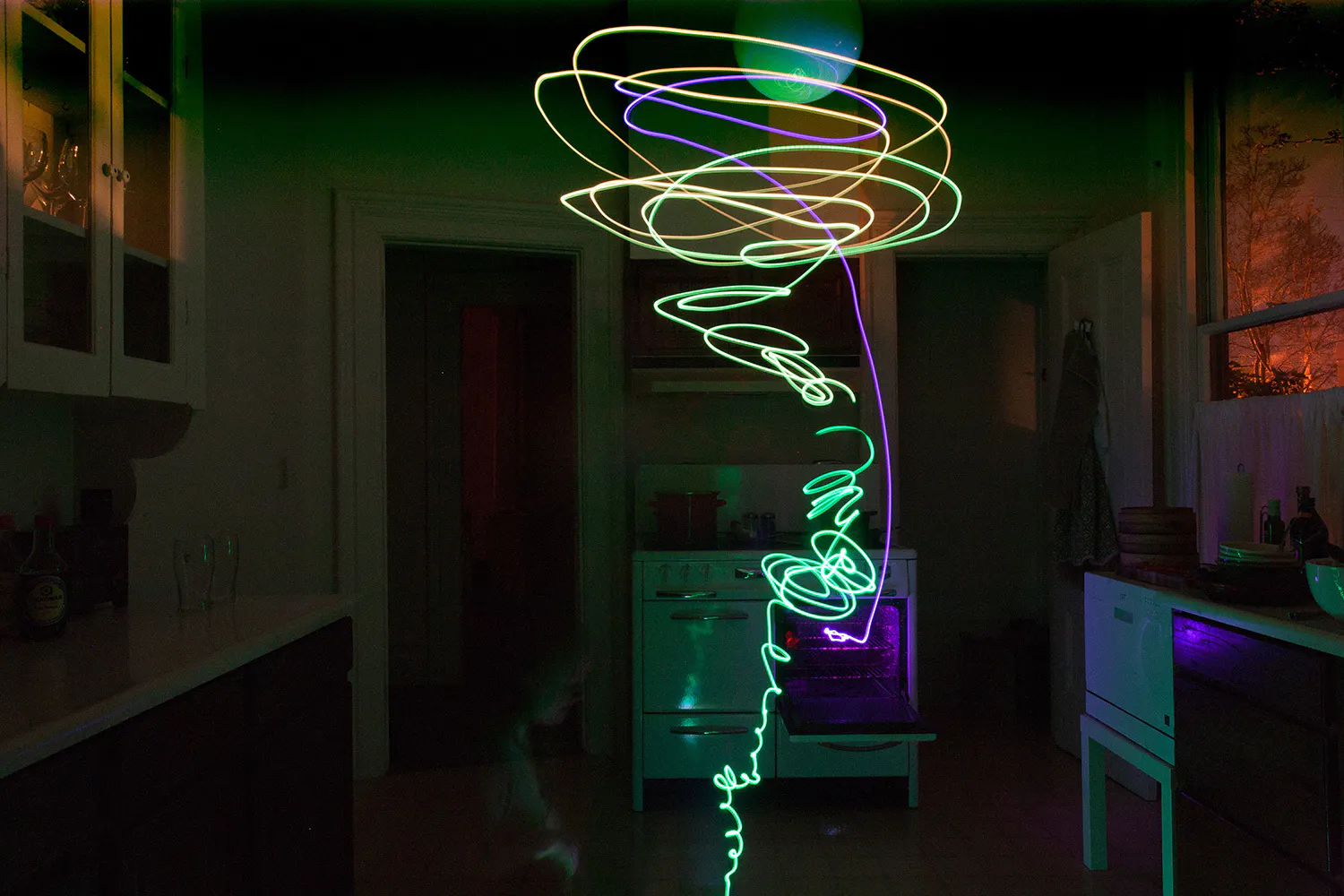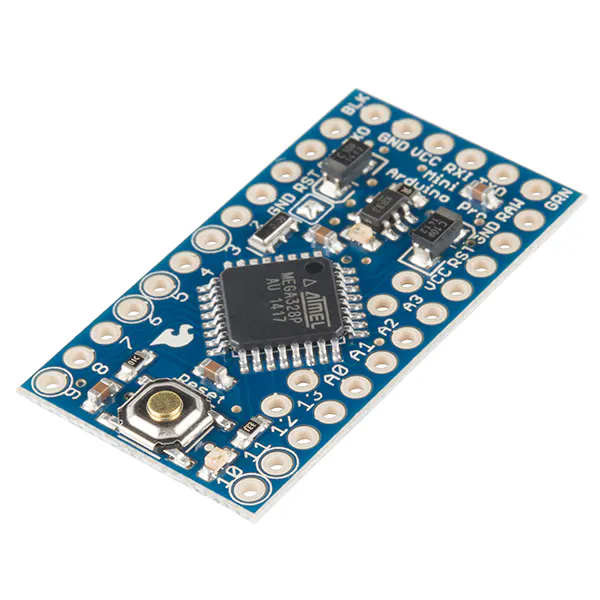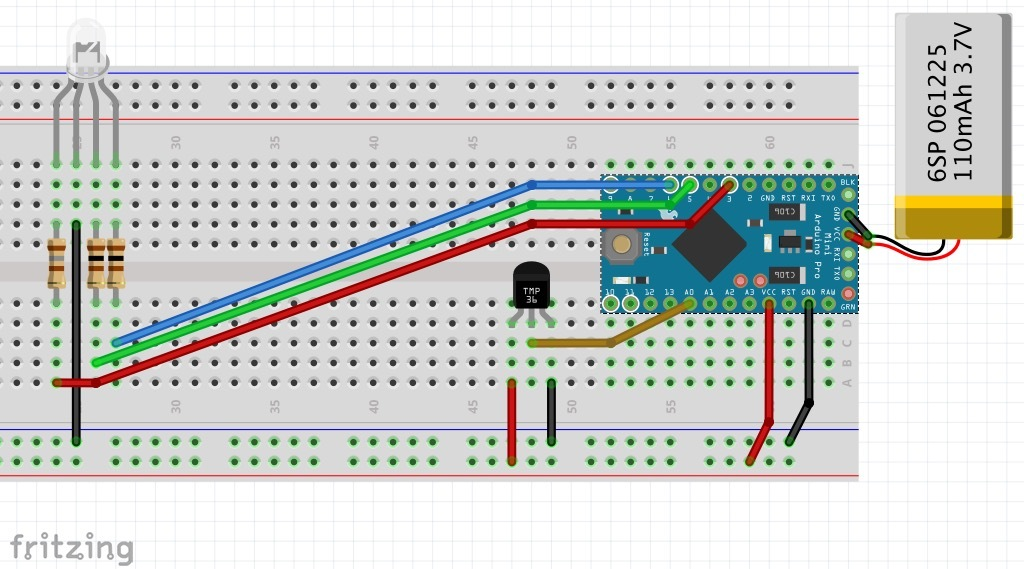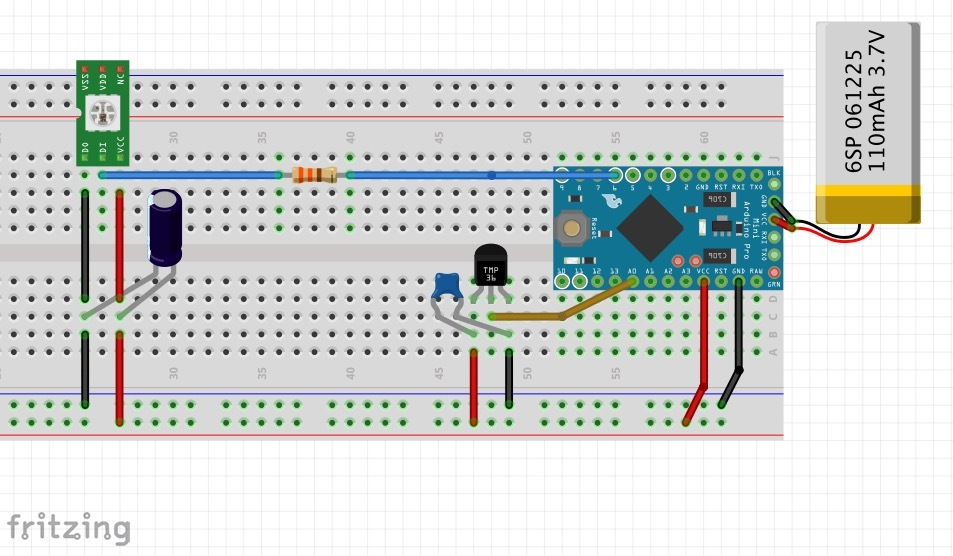Project in progress# Weather Wand

Magic wand to paint with color light based on temperature.

• 17,295 views
• 32 respects

## Components and suppliesSparkFun Arduino Pro Mini 328 - 3.3V/8MHz
×1
 TMP36 sensor
×1
 NeoPixel RGB LED
×1
 100 Ohm resistor (Green and Blue channel)
×2
 150 Ohm resistor (red channel)
×1
 110mah 3.7v lipo battery
×1

The wondrous magical weather wand casts an enchanting spell of light. Away to make the invisible visible.

In less magical terms, I have an Arduino pro mini with a temperature sensor (currently a TMP36) and a RGB LED. The Arduino checks temperatures and sets the color of the light based on the temperature.

The early version of this used a temp to color table (like the temperature map project) that would for example set to the light yellow if above 70º or to blue if below 30º. However it would be pretty unlikely to encounter that wide of a temperature range within a space so instead of re-tuning the table for every location I wanted to have it set it's own range.

The solution was to use statistics! It takes and averages the temperatures it sees and sets the color to normal (green) based on that average. The standard deviation (ie max/min range) is set based on the variation the sensor picks up. The color changes based not on a specific temp value but the number of standard deviations from that average. ie: 2+ stddev is red -2 stddev is purple, etc.

Testing the temperature variations around the room

Making of

Future plans:

Would like to make a nice magic wand case, maybe fimo clay or something.

Experimenting with timing and other sensors to get a faster response rate and better sensitivity to change.

Might work on gradients between values instead of 'click-stops' for color change values.

Add a small capacitor to the TMP36 power input to stabilize it. (Currently stabilizing in code and it is slowing response times)

## Code

##### wx_wand_1.txtC/C++
Yummy code snacks
```/*
The Weather Wand!

A small wand that lights up at the tip based on if it is hot or cold.

Hot and cold are determined by the standard deviation from the average of the 50 most recent temperature samples.

Hardware: An Arduino pro mini with a TMP36 temp sensor and an common cathode RGB LED.

By: Dan Fein with great super math help from John Celenza.

*/

//________________Setup________________________

int DEBUG = 1;      // DEBUG counter; if set to 1, will write values back via serial

// Init the Pins used for PWM
const int redPin = 3;
const int greenPin = 5;
const int bluePin = 6;

//Temp sensor stuff
int sensorPin = 0; //TMP36
float tempF;
float tempC;

// Vars for the color output
int redVal = 0;
int grnVal = 0;
int bluVal = 0;

//delay var
int del = 100;

//statistics
int max_samples = 50;
int temperature_samples;
int n_temperature_samples = 0;
int i_temperature_samples = 0;
float stddev_temperature = 5;
float avg_temperature = 50;
float tempF_recent = 0;

//________________Run Time________________________

void setup()
{
pinMode(redPin, OUTPUT);
pinMode(greenPin, OUTPUT);
pinMode(bluePin, OUTPUT);

if (DEBUG) {         // If we want to see values for debugging...
Serial.begin(9600);  // ...set up the serial ouput
Serial.println("Starting");
}
}

void loop()
{
getTemp();

temperature_samples[i_temperature_samples] = tempF;
i_temperature_samples++;
if (n_temperature_samples < max_samples) {
n_temperature_samples++;
}
if (i_temperature_samples >= max_samples) {
i_temperature_samples = 0;
}

computeStdDev();

tempColor();

delay(del);
}

// ---------------- Function Fun --------------------

void getTemp()
{
//getting the voltage reading from the temperature sensor

// converting that reading to voltage, for 3.3v arduino use 3.3, for 5v use 5
float voltage = reading * 3.3;
voltage /= 1024.0;

// now kick out the temperature
tempC = (voltage - 0.5) * 100 ;  //converting from 10 mv per degree with 500 mV offset
//to degrees ((voltage - 500mV) times 100)

// Convert to Fahrenheit
tempF = (tempC * 9.0 / 5.0) + 32.0;

if (DEBUG)   // If we want to print it all to console...
{
Serial.println("++++++++++++++");
Serial.print(voltage); Serial.println(" volts");
Serial.print(tempC); Serial.println(" degrees C");
Serial.print(tempF); Serial.println(" degrees F");
Serial.print(tempF_recent); Serial.println(" degrees F (recent)");

Serial.println("--------------");
}

}

void computeStdDev() {
int i;
avg_temperature = 0;
for (i = 0; i < n_temperature_samples; i++){
avg_temperature = avg_temperature + temperature_samples[i];
}
avg_temperature = avg_temperature / n_temperature_samples;

stddev_temperature = 0;
for (i = 0; i < n_temperature_samples; i++){
stddev_temperature = stddev_temperature + (temperature_samples[i] - avg_temperature) * (temperature_samples[i] - avg_temperature);
}

stddev_temperature = stddev_temperature / n_temperature_samples;
stddev_temperature = sqrt(stddev_temperature);

int start_i = i_temperature_samples - 5;
if (start_i < 0) {
start_i = 0;
}

if (i_temperature_samples - start_i > 0){

tempF_recent = 0;
for (i = start_i; i < i_temperature_samples; i++) {
tempF_recent = tempF_recent + temperature_samples[i];
}
tempF_recent /= (i_temperature_samples - start_i);

} else {
tempF_recent = tempF;
}

if (DEBUG)   // If we want to print it all to console...
{
Serial.print("n_temperature_samples: ");
Serial.println(n_temperature_samples);
Serial.print("avg_temperature: ");
Serial.println(avg_temperature);
Serial.print("temperature: ");
Serial.println(tempF);
Serial.print("i: ");
Serial.println(i_temperature_samples);
Serial.print("stddev_temperature: ");
Serial.println(stddev_temperature);
}
}

//Set the RGB color based on standard deviation distance from average temp
void tempColor()
{
float diff = (tempF_recent - avg_temperature) / stddev_temperature;

if(diff < -2.5) {
setColor(255, 0, 255);
} else if (diff < -2.0) {
setColor(0, 100, 255);
} else if (diff < -1.5) {
setColor(0, 157, 200);
} else if (diff < -1.0) {
setColor(150, 206, 150);
} else if (diff < -0.5) {
setColor(0, 200, 50);
} else if (diff < 0.0) {
setColor(0, 255, 1);
} else if (diff < 0.5) {
setColor(150, 255, 0);
} else if (diff< 1.0) {
setColor(200, 200, 0);
} else if (diff < 1.5) {
setColor(255, 100, 0);
} else if (diff < 2.0) {
setColor(255, 10, 0);
} else {
setColor(100, 100, 100); //out of scope color
}

if (DEBUG)   // Standard deviation visualizer
{
Serial.print("diff: ");
Serial.println(diff);

if(diff < -2.5) {
Serial.println("X----0+++++");
} else if (diff < -2.0) {
Serial.println("-X---0+++++");
} else if (diff < -1.5) {
Serial.println("--X--0+++++");
} else if (diff < -1.0) {
Serial.println("---X-0+++++");
} else if (diff < -0.5) {
Serial.println("----X0+++++");
} else if (diff < 0.0) {
Serial.println("-----0+++++");
} else if (diff < 0.5) {
Serial.println("-----0X++++");
} else if (diff< 1.0) {
Serial.println("-----0+X+++");
} else if (diff < 1.5) {
Serial.println("-----0++X++");
} else if (diff < 2.0) {
Serial.println("-----0+++X+");
} else {
Serial.println("X----0++++X");
}
}
}

//Send the RGB value to the color pins
void setColor(int r, int g, int b) //0-255 input
{
// plug it in
int redVal = r;
int grnVal = g;
int bluVal = b;

analogWrite(redPin, redVal);   // Write current values to LED pins
analogWrite(greenPin, grnVal);
analogWrite(bluePin, bluVal);

if (DEBUG)   // If we want to print it all to console...
{
Serial.print(redVal); Serial.print(" R ");
Serial.print(grnVal); Serial.print(" G ");
Serial.print(bluVal); Serial.println(" B ");
Serial.println("--------------");
}
}
```
##### file_15302.txtC/C++
BONUS! - Code to run NeoPixels instead of a single RGB LED, also on GitHub above
```/*
The Weather Wand!

A small wand that lights Neo Pixels (addressable RGB LEDs) at the tip in response to temperature .

Hot and cold are determined by the standard deviation from the average of the 50 most recent temperature samples.

Hardware: An Arduino pro mini with a TMP36 temp sensor and addressable RGB LEDs.

By: Dan Fein with great super math help from John Celenza.

*/

//________________Setup________________________

int DEBUG = 1;      // DEBUG counter; if set to 1, will write values back via serial

// NeoPixel setup
#define PIN 6

// Parameter 1 = number of pixels in strip
// Parameter 2 = Arduino pin number (most are valid)
// Parameter 3 = pixel type flags, add together as needed:
//   NEO_KHZ800  800 KHz bitstream (most NeoPixel products w/WS2812 LEDs)
//   NEO_KHZ400  400 KHz (classic 'v1' (not v2) FLORA pixels, WS2811 drivers)
//   NEO_GRB     Pixels are wired for GRB bitstream (most NeoPixel products)
//   NEO_RGB     Pixels are wired for RGB bitstream (v1 FLORA pixels, not v2)

// IMPORTANT: To reduce NeoPixel burnout risk, add 1000 uF capacitor across
// pixel power leads, add 300 - 500 Ohm resistor on first pixel's data input
// and minimize distance between Arduino and first pixel.  Avoid connecting
// on a live circuit...if you must, connect GND first.

//Temp sensor setup
int sensorPin = 0; //TMP36
float tempF;
float tempC;

//delay var
int del = 80;

//statistics
int max_samples = 50;
int temperature_samples;
int n_temperature_samples = 0;
int i_temperature_samples = 0;
float stddev_temperature = 5;
float avg_temperature = 50;
float tempF_recent = 0;

//________________Run Time________________________

void setup()
{
strip.begin();
strip.show(); // Initialize all pixels to 'off'
strip.setBrightness(50); // Turn down for what 0-255

if (DEBUG) {         // If we want to see values for debugging...
Serial.begin(9600);  // ...set up the serial ouput
Serial.println("Starting");
}
}

void loop()
{
getTemp();

temperature_samples[i_temperature_samples] = tempF;
i_temperature_samples++;
if (n_temperature_samples < max_samples) {
n_temperature_samples++;
}
if (i_temperature_samples >= max_samples) {
i_temperature_samples = 0;
}

computeStdDev();

tempColor();

delay(del);
}

// ---------------- Function Fun --------------------

void getTemp()
{
//getting the voltage reading from the temperature sensor

// converting that reading to voltage, for 3.3v arduino use 3.3, for 5v use 5
float voltage = reading * 3.3;
voltage /= 1024.0;

// now kick out the temperature
tempC = (voltage - 0.5) * 100 ;  //converting from 10 mv per degree with 500 mV offset
//to degrees ((voltage - 500mV) times 100)

// Convert to Fahrenheit
tempF = (tempC * 9.0 / 5.0) + 32.0;

if (DEBUG)   // If we want to print it all to console...
{
Serial.println("++++++++++++++");
Serial.print(voltage); Serial.println(" volts");
Serial.print(tempC); Serial.println(" degrees C");
Serial.print(tempF); Serial.println(" degrees F");
Serial.print(tempF_recent); Serial.println(" degrees F (recent)");
Serial.println("--------------");
}

}//end

void computeStdDev() {
int i;
avg_temperature = 0;

for (i = 0; i < n_temperature_samples; i++){
avg_temperature = avg_temperature + temperature_samples[i];
}

avg_temperature = avg_temperature / n_temperature_samples;

stddev_temperature = 0;
for (i = 0; i < n_temperature_samples; i++){
stddev_temperature = stddev_temperature + (temperature_samples[i] - avg_temperature) * (temperature_samples[i] - avg_temperature);
}

stddev_temperature = stddev_temperature / n_temperature_samples;
stddev_temperature = sqrt(stddev_temperature);

int start_i = i_temperature_samples - 5;
if (start_i < 0) {
start_i = 0;
}

if (i_temperature_samples - start_i > 0){

tempF_recent = 0;
for (i = start_i; i < i_temperature_samples; i++) {
tempF_recent = tempF_recent + temperature_samples[i];
}
tempF_recent /= (i_temperature_samples - start_i);

} else {
tempF_recent = tempF;
}

if (DEBUG)   // If we want to print it all to console...
{
Serial.print("n_temperature_samples: ");
Serial.println(n_temperature_samples);
Serial.print("avg_temperature: ");
Serial.println(avg_temperature);
Serial.print("temperature: ");
Serial.println(tempF);
Serial.print("i: ");
Serial.println(i_temperature_samples);
Serial.print("stddev_temperature: ");
Serial.println(stddev_temperature);
}
}//end

//Set the RGB color based on standard deviation distance from average temp
void tempColor()
{
float diff = (tempF_recent - avg_temperature) / stddev_temperature;

if(diff < -2.5) {
setColor(strip.Color(255, 0, 255));
} else if (diff < -2.0) {
setColor(strip.Color(0, 100, 255));
} else if (diff < -1.5) {
setColor(strip.Color(0, 157, 200));
} else if (diff < -1.0) {
setColor(strip.Color(150, 206, 150));
} else if (diff < -0.5) {
setColor(strip.Color(0, 230, 50));
} else if (diff < 0.0) {          // 0 = Avg
setColor(strip.Color(0, 255, 1));
} else if (diff < 0.5) {
setColor(strip.Color(50, 230, 0));
} else if (diff< 1.0) {
setColor(strip.Color(200, 200, 0));
} else if (diff < 1.5) {
setColor(strip.Color(255, 100, 0));
} else if (diff < 2.0) {
setColor(strip.Color(255, 50, 0));
} else {
setColor(strip.Color(255, 0, 0)); //max out color
}

if (DEBUG)   // Standard deviation visualizer
{
Serial.print("diff: ");
Serial.println(diff);

if(diff < -2.5) {
Serial.println("X----0+++++");
} else if (diff < -2.0) {
Serial.println("-X---0+++++");
} else if (diff < -1.5) {
Serial.println("--X--0+++++");
} else if (diff < -1.0) {
Serial.println("---X-0+++++");
} else if (diff < -0.5) {
Serial.println("----X0+++++");
} else if (diff < 0.0) {
Serial.println("-----0+++++");
} else if (diff < 0.5) {
Serial.println("-----0X++++");
} else if (diff< 1.0) {
Serial.println("-----0+X+++");
} else if (diff < 1.5) {
Serial.println("-----0++X++");
} else if (diff < 2.0) {
Serial.println("-----0+++X+");
} else {
Serial.println("X----0++++X");
}
}
} //end

//Send the RGB value to the pixels
void setColor(uint32_t c) {
for(uint16_t i=0; i<strip.numPixels(); i++) {
strip.setPixelColor(i, c);
strip.show();
}
} //end
```
##### Github
And of course on GitHub

## Schematics1000uF cap on pixel power, 3-500 ohm resistor on data, also added a .1uF cap to stabilize the TMP36#### Author

• Math help by John Celenza

March 29, 2015

#### Members who respect this project

See similar projects
you might like

#### WiFi Weather Station

Project in progress by Dan Fein

• 42,388 views
• 70 respects

#### Pocket Weather Station Using Arduino

Project tutorial by Tech Nuttiez

• 3,313 views
• 7 respects

#### DIY Arduino Remote for TV

Project tutorial by Arnov Sharma

• 8,984 views
• 8 respects

#### Playing "Flappy Bird" on an LED Matrix

Project tutorial by Arduino “having11” Guy

• 6,693 views
• 1 comment
• 20 respects

#### smart robot

Project in progress by Team Arduino Arab

• 5,467 views
• 1 comment
• 10 respects

by Iain

• 28,871 views